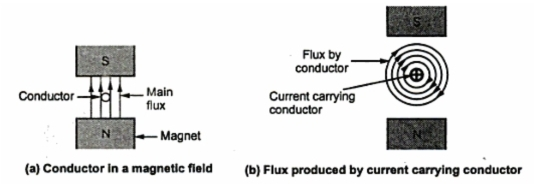### D.C. Motors

1. Introduction
A motor is a device which converts an electrical energy into the mechanical energy . The energy conversion process is exactly opposite to that involved in a d.c. generator. In a generator the input mechanical energy is supplied by a prim mover while in a d.c. motor, input electrical energy is supplied by a d.c. supply. The construction of a d.c. machine is same whether it is a motor or a generator.
2. Principle of Operation of a D.C. Motor
The principle of operation of a d.c. motor can be stated in a single statement as 'when a current carrying conductor is placed in a magnetic field' it experiences a mechanical force'. In a practical d.c. motor, field winding produces a required magnetic field while armature conductors play a role of a current carrying conductors and hence armature conductors experience a force. As a conductors are placed in the slots which are in the periphery, the individual force experienced by the conductors acts as a twisting or turning force on the armature which is called a torque. The torque is the product of force and the radius at which this force acts. So overall armature experiences a torque and starts rotating. Let us study this motoring action in detail.
Consider a single conductor placed in a magnetic field as shown in the Fig .1(a). The magnetic field is produced by a permanent magnet but in a practical d.c. motor it is produced by the field winding when it carries a current.Fig.  1
Now this conductor is excited by a separate supply so that it carries a current in a particular direction. Consider that it carries a current away from an observe as shown in the Fig. 1(b). Any current carrying conductor produces its own magnetic field around it. hence this conductor also produces its own flux, around. The direction of this flux can be determined by right hand thumb rule. For direction of current considered, the direction of flux around a conductor is clockwise. For simplicity of understanding, the main flux produced by the permanent magnet is not shown in the Fig. 1(b).
Now there are two fluxes present,
1. The flux produced by the permanent magnet called flux.
2. The flux produced by the current carrying conductor.
There are shown in the Fig.2(a). Form this, it is clear that on one side of the conductor, both the fluxes are in same direction. In this case, on the left of the conductor there is gathering of the flux lines as two fluxes help each other. As against this, on the right of the conductor, the two fluxes are in opposite direction and hence try to cancel each other. Due to this, the density of the flux lines in this area gets weakened. So on the left, there exists high flux density area while on the right of the conductor there exists low flux density area as shown in the Fig. 2(b).Fig. 2

This flux distribution around the conductors acts like a stretched rubber band under tension. This exerts a mechanical force on the conductor which acts from high flux density area towards low flux density area. i.e. from left to right for the case considered as shown in the Fig. 2(b).Fig.  3

Key point : In the practical d.c. motor, the permanent magnet is replaced by a field winding which produces the required flux called main flux and all the armature conductors, mounted on the periphery of the armature drum, get subjected to the mechanical force. Due to this, overall armature experiences a twisting force called torque and armature of the motor starts rotating.

Related articles :
Sponsored links